# IS trapezoid

Isosceles trapezoid arm measured 35 cm. Height is 30 cm and middle segment is 65 cm. Determine length of its bases.

Correct result:

a =  83.03 cm
c =  46.97 cm

#### Solution: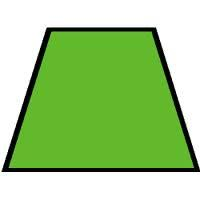We would be pleased if you find an error in the word problem, spelling mistakes, or inaccuracies and send it to us. Thank you!Tips to related online calculators
Do you have a linear equation or system of equations and looking for its solution? Or do you have quadratic equation?

#### You need to know the following knowledge to solve this word math problem:

We encourage you to watch this tutorial video on this math problem:

## Next similar math problems:

• Drainage channel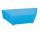The cross section of the drainage channel is an isosceles trapezoid whose bases have a length of 1.80 m, 0.90 m and arm has length 0.60 meters. Calculate the depth of the channel.
• Parcelparcel has a rectangular shape of a trapezoid with bases 12 m and 10 m and a height 8 m. On parcel was built object with a footprint an isosceles triangle shape with side 4 m and height three-quarters of a meter. What is the area of unbuild parcel?
• Trapezoid v3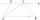The isosceles trapezoid has the angle at a firsst base 40 degrees less than the angle at second base. How large angles has this trapezoid ?
• StairwayStairway has 20 steps. Each step has a length of 22 cm and a height of 15 cm. Calculate the length of the handrail of staircases if on the top and bottom exceeds 10 cm.
• Complementary angles 2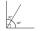Two complementary angles are (x+4) and (2x - 7) find the value of x
• WindbreakA tree at a height of 3 meters broke in the windbreak. Its peak fell 4.5 m from the tree. How tall was the tree?
• Right triangleRight triangle ABC with side a = 19 and the area S = 95. Calculate the length of the remaining sides.
• Horizontal distance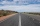The road has a gradient of 8%. How many meters will the road rise on a horizontal distance of 400m?
• Tickets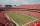On the football tournament ticket cost 45 Kc for standing and 120kč for sitting. Sitting spectators was 1/3 more than standing. The organizers collected a total 12 300 Kc. How many seated and standing seats (spectators)?
• Percentage reduction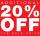Reducing the unknown number by 19% get number 700.7 Determine unknown number.
• Center traverseIt is true that the middle traverse bisects the triangle?
• EquationSolve the equation: 1/2-2/8 = 1/10; Write the result as a decimal number.
• CandyPeter had a sachet of candy. He wanted to share with his friends. If he gave them 30 candies, he would have 62 candies. If he gave them 40 candies, he would miss 8 candies. How many friends did Peter have?
• Heating plant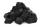Workers dump imported coke at heating plant. On the first day used half of the amount, the second day three quarters the rest and on the third day left 120 tons. How much coke they dump at heating plant?
• Simple equationSolve for x: 3(x + 2) = x - 18
• Unknown numberIdentify unknown number which 1/5 is 40 greater than one tenth of that number.
• Fifth of the numberThe fifth of the number is by 24 less than that number. What is the number?